###Microsoft Excel is a magical program that most of the office employees utilize to easily perform complex calculation and make the work done easily. At first glance, it might look like a program with only tables and slots for entering data, but this description is not enough to know about the real capabilities of this program.

Excel can do anything from managing your office accounts to managing the data required for the whole country but for that, you just need to know complete about it. Here in this tutorial, we are describing Excel troubleshooting formula that helps you to easily analyze data.

Let’s take a look:

### List Of Some Commonly Used Functions

Here learn the powerful Excel troubleshooting tricks for analysing data.

### Simple Calculations

In order to solve pretty complex calculations, Excel can help you do simple arithmetic like, adding, multiplying, subtracting, or dividing any of your data.

• To add, use the + sign.
• For subtracting, use the – sign.
• To multiply, use the * sign.
• To divide, use the / sign.

You need to use parenthesis in order to ensure certain calculations first. For example, you need to solve this (5+5*5), the 2nd and 3rd 5 were multiplied together before adding the additional 5. However, if we write in this way (5+5)*5, then the 1st and 2nd would be added together first.

Like this, if you want to take out the average of a set of numbers, then you can use the formula: AVERAGE(Cell Range). If you want, to sum up, columns of numbers, then you can use the formula: SUM(Cell Range).

### Creating Charts:

Building a graph or chart in Excel requires nothing more than selecting the range of data you wish to chart and press F11. This will make Excel chart in default chart style but you can change it by selecting different chart style. If you prefer the chart to be on the same worksheet where the data is then instead of pressing F11 press ALT+F1.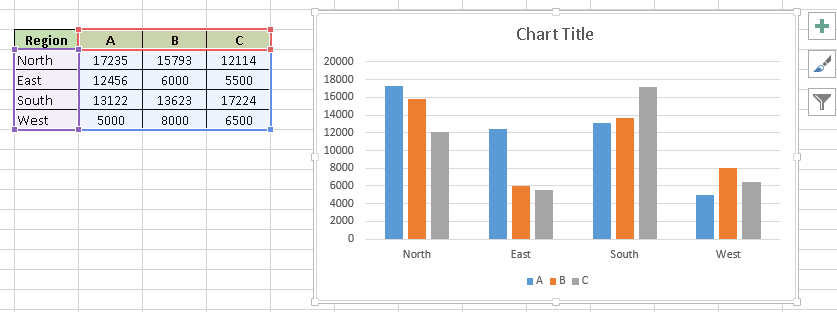### Vlookup

Vlookup is the power tool that every Excel user should know. It helps you to group data that is scattered across different sheets and workbooks and bring those sheets into a central location to create reports and summaries. It is more helpful when you need to find information in large data tables such as inventory lists.Syntax:

Syntax: =VLOOKUP(Key to lookup, Source_table, a column of the source table, are you ok with a relative match?)

For above problem, you need to write a formula in cell “F4” as =VLOOKUP(B4, \$H\$4:\$L\$15, 5, 0) and thus it will return the city name for customer id 1 and post that copy this formula for all Customer ids.

But remember don’t forget to close the range of the second table using “\$” sign, as it is the most common error that happens while copying down the formula.

### CONCATINATE():

It is very helpful excel function as by using this you can combine text from two or more cells. Suppose that you want to create a URL based on an input of host name and request path.

Syntax: =Concatenate(Text1, Text2,…..Textn)

So to do this, use formula = concatenate(B3,C3) and copy it.

Tip: you can also write the formula as “= B3&C3” instead of writing full “concatenate” formula.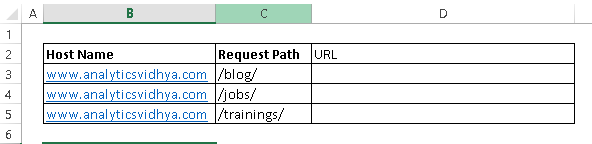### LOWER(), UPPER() and PROPER()–

These three functions help you to change the text to lower, upper case respectively.

Syntax: =Upper(Text)/ Lower(Text) / Proper(Text)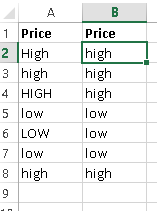In data analysis workaround, these are very helpful in converting classes of different case to a single one else these are considered as different classes of the given features.

### Remove duplicate values

It removes the given values from given table based on selected columns. Means if you select two columns then it searches for duplicate value having a combination of both columns data.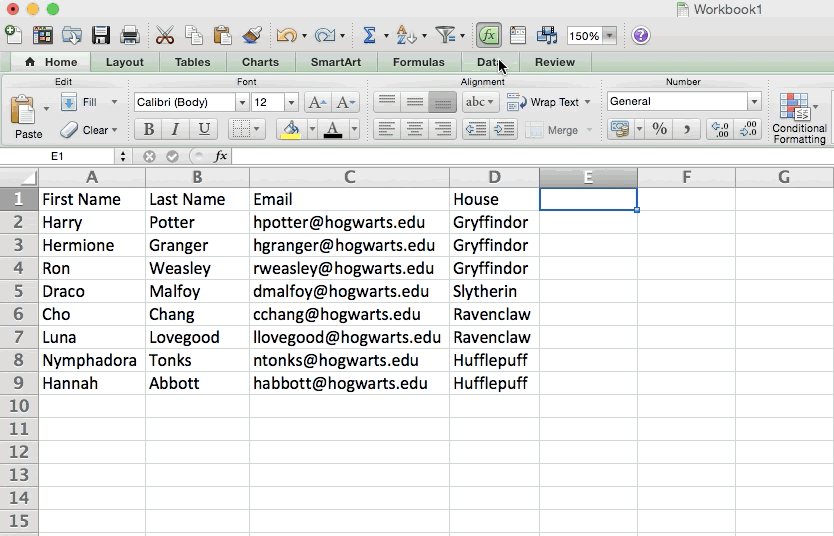As you can see that A001 and A002 have duplicate value but if you select both columns “ID” and “Name” then you have only one duplicate value left (A002, 2). To remove off the duplicate data: Select data –> Go to Data ribbon –> Remove Duplicates

### Pivot Tables

Pivot tables are basically used to recognize data in a spreadsheet. They won’t change the data that you have but they add up values and compare different information in your spreadsheet, depending on what you did like them to do.

To create the pivot table, go to data > Pivot Table. Excel will automatically populate your pivot table but you can always make changes in the order of the data.  Excel will automatically populate your Pivot table, but you can always change around the order of the data. Then, you have four options to choose from:

Report filter:

This allows you to search any particular rows in your data set. For example: suppose we need to create a filter by house then you need to select only include the student in Gryffindor instead of all students.

Column labels: this could be your headers in the data set.

Row labels: this could be your rows in the data set. Both row and column labels contain data from your columns (e.g. First Name can be dragged wither to either the Row or Column label – it totally depends on how you want to see the data.

Value:

This section allows you to look at your data differently. Instead of pulling any numeric value, you can count, sum, average, max, min, count numbers, or do a few other manipulations with your data. In fact by default when you drag a field to a value, it always makes a count.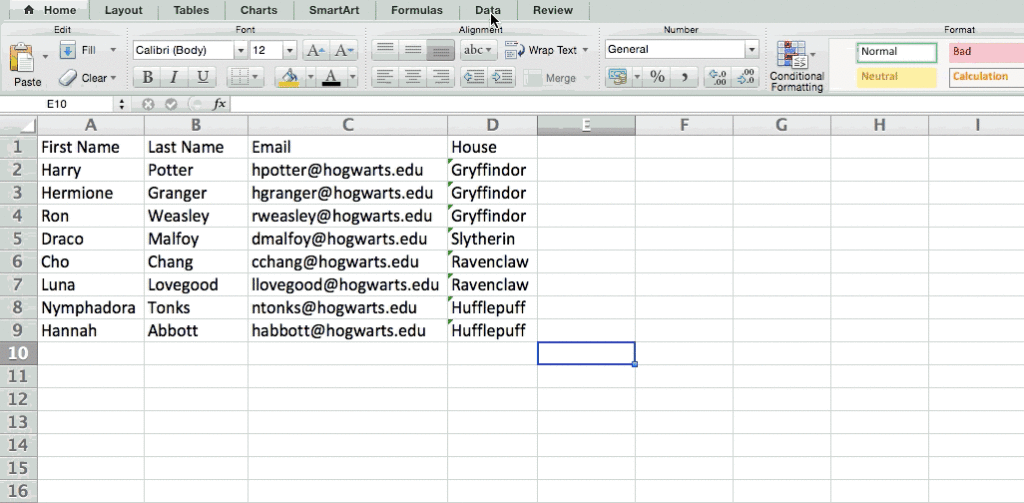### Precedents & Dependents

If you are dealing with a huge spreadsheet which contains lots of functions and formulas then it will be a terrible need to know the precedents and dependents of function/formula. Excel 2010’s tracking Precedents and Tracking Dependents features that would let you know which are the precedents and dependents of the selected cell containing formula or functions. This feature tells you from where the function is taking values and how it is dependents depicting directed arrows towards the concerned fields.

Suppose, you have included a spreadsheet in which you have to find out database average and sum of students’ record through DAVERAGE and DSUM function.

For finding the Dependents and Precedents of the formula, select the formula cell and navigate to formula tabs, click Trace Precedents.

It will instantly, show the blue lines with arrowheads depicting all Precedents formula cells.

Now, check the Dependents of value in Status field, now select any cell in the status field and from the formula tab, choose Trace Dependents. It will depict all the dependents of the formula cell, as shown below.

### IF Statement

Sometimes you don’t want to count the number of times a value appears. Instead, you want to input different information into a cell if there is the corresponding cell with that information

For example, as here in the figure, if you want to add 10 points to everyone who belongs in the Gryffindor house. Instead of manually typing in 10’s next to each Gryffindor student’s name, you can use the IF THEN excel formula saying that if the student is in Gryffindor, then they should get 10 points.

The formula: IF(logical_test, value_if_true, value of false)

Example Shown Below: =IF(D2=”Gryffindor”,”10″,”0″)

In general; term the formula would be IF (Logical test, value of true, value of false) let’s dig into each of these variables.

Logical test: the logical test is the “if” part of the statement. In this case, the logic is D2=”Gryffindor” we want to make this clear that the cell corresponding with the student says “Gryffindor.”

Value_if_True: this is what you need, you want the cell to show if the value is true. Suppose you want the cell to show “5” to indicate that the student was awarded the 5 points. Instead of numbers if you want the text then only use quotations.

Value_if_False: this is what you want the cell to show if the value is false. For such cases, for any student not in Gryffindor, you want that the cell should show “0” to show 0 points. Only use quotation marks if you want the result to be text instead of a number.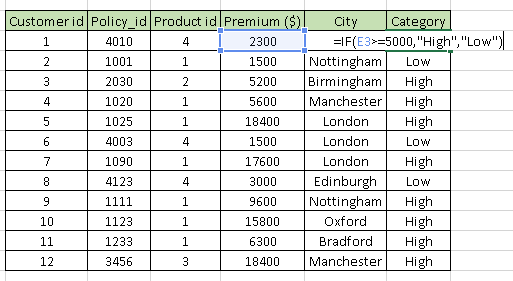### Text to Columns

In the Excel function, there is also an option to split out information contained in one cell into two different cells. Suppose you want to take out someone’s company name through their email address or perhaps you want to separate someone’s full name into a first and last name for your email marketing templates.

To do this, first, highlight the column that you want to split. After then, go to the Data tab and select “Text to Columns.”  A module will appear with the additional information.

First, you need to select either “Delimited” or “Fixed Width.”

“Delimited” means you want to break up the column based on characters such as commas, spaces, or tabs.

“Fixed width” is being used when you want to select the exact location on all the columns which you want the split to occur.

In the given example case, let’s select “Delimited” so you can separate the full name into first name and last name.

### COUNTIF Function

Instead of manual counting how often a certain value or number appears, so let’s Excel do the work for you. With the COUNTIF function, Excel counts the number of times a number or word appears in any range of cells.

For example, let’s say I want to count the number of times the word “Gryffindor” appears in the dataset.

The formula: =COUNTIF(range, criteria)

The formula with variables from our example below: =COUNTIF(D:D,”Gryffindor”)

## Automatic Resolution:

There are many cases due to which the Excel files get corrupted, damaged and start showing error, so if you are the one facing the excel file corruption then, in this case, make use of the MS Excel File Repair Tool this is the best tool to repair the excel file as well as recovers data. It is designed by professional with highly algorithms and deep scanning technique that can easily repair and retrieve corrupted Excel file without any issue.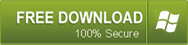### End Notes

Undoubtedly, Excel is arguably one of the programs that ever made and it has remained the gold standard for nearly all business worldwide. Whether you are a newbie or a power user, there is always something to learn. So hopefully all the have mentioned Excel functions will surely help you in using your excel spreadsheet more enhance.

Good Luck!!!11 Simple Yet Powerful Excel Troubleshooting Tricks for Analyzing Data
SummaryArticle Name
11 Simple Yet Powerful Excel Troubleshooting Tricks for Analyzing Data
Description
Using Excel then here follow the powerful Excel troubleshooting tricks for data analyzing, these are easy even a novice users can use it…
Author
Publisher Name
Repair MS Excel Blogs
Publisher Logo
• 5
Shares